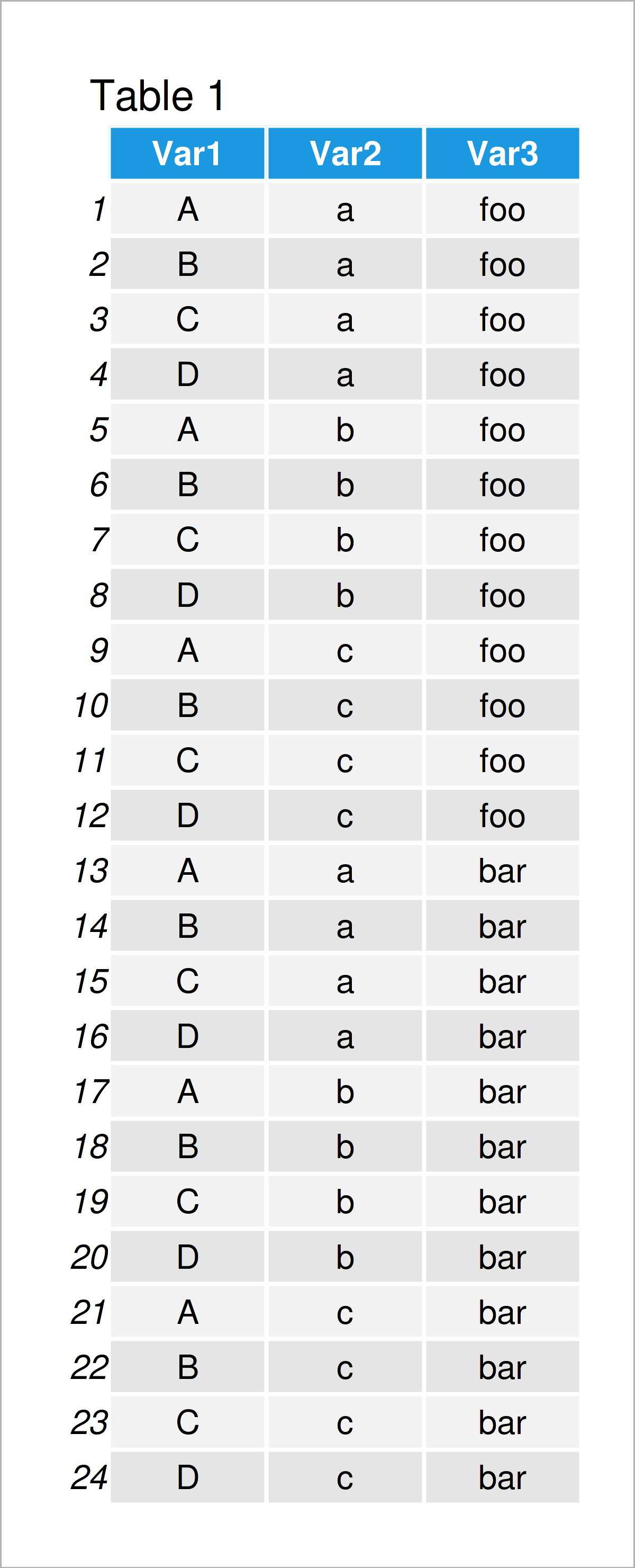# Cartesian Product in R (2 Examples)

This page illustrates how to get the Cartesian product in the R programming language.

Let’s jump right to the examples:

## Construction of Example Data

We’ll use the following data as basement for this tutorial:

```x <- LETTERS[1:4]                   # Create first example vector
x                                   # Print first example vector
#  "A" "B" "C" "D"```
```y <- letters[1:3]                   # Create second example vector
y                                   # Print second example vector
#  "a" "b" "c"```
```z <- c("foo", "bar")                # Create third example vector
z                                   # Print third example vector
#  "foo" "bar"```

Have a look at the previous outputs of the RStudio console. It shows that we have created three vector objects called x, y, and z. Each of these vectors contains multiple character letters and strings.

## Example 1: Get Cartesian Product Using expand.grid() Function

This example shows how to calculate the Cartesian product of several vectors using the expand.grid function.

Consider the following R code:

```data_cp1 <- expand.grid(x, y, z)    # Apply expand.grid function
data_cp1                            # Print Cartesian product```Table 1 illustrates the output of the previous R syntax. As you can see, we have created a data frame containing a set of all ordered pairs of our vectors.

## Example 2: Get Cartesian Product Using crossing() Function of tidyr Package

In Example 2, I’ll illustrate how to use the tidyr package to find the Cartesian product.

If we want to use the functions of the tidyr package, we first have to install and load tidyr:

```install.packages("tidyr")           # Install tidyr package

Next, we can apply the crossing function of the tidyr package to identify the Cartesian product:

```data_cp2 <- crossing(x, y, z)       # Apply crossing function
data_cp2                            # Print Cartesian product
# # A tibble: 24 x 3
#    x     y     z
#    <chr> <chr> <chr>
#  1 A     a     bar
#  2 A     a     foo
#  3 A     b     bar
#  4 A     b     foo
#  5 A     c     bar
#  6 A     c     foo
#  7 B     a     bar
#  8 B     a     foo
#  9 B     b     bar
# 10 B     b     foo
# # ... with 14 more rows```

The previous output is a tibble containing the Cartesian product of our three input vectors.

## Video & Further Resources

I have recently released a video on my YouTube channel, which illustrates the content of this article. You can find the video below.

Besides that, you might read the related articles on my website.

This article has demonstrated how to find a data matrix containing the Cartesian product of vector objects in the R programming language.

In this tutorial, we have used three vectors as input. However, please note that we could use the same kind of R code to calculate the set of ordered pairs of more input vectors as well (e.g. two, four, five…).